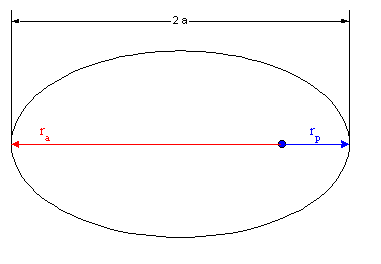# Energy principle and circular/ellipse orbits

Question is as follows:
How much energy do i need when i move an object with a mass M from a circular orbit with a radius of R_1 to an ellipse orbit with aphelion radius of R_2.

I'm assuming energy principle is the way to go here but it leads to a question i'd like someone to help me out with.

Do i need to assume the perihelion radius of the new orbit (aphelion radius R_2) is the circular orbit's radius R_1?

gneill
Mentor
Question is as follows:
How much energy do i need when i move an object with a mass M from a circular orbit with a radius of R_1 to an ellipse orbit with aphelion radius of R_2.

I'm assuming energy principle is the way to go here but it leads to a question i'd like someone to help me out with.

Do i need to assume the perihelion radius of the new orbit (aphelion radius R_2) is the circular orbit's radius R_1?

You can assume anything you like; The answer you get will depend upon your assumptionsThe Total Specific Mechanical Energy, $\xi$, of an orbit is inversely proportional to the size of its major axis. Thus $\xi = -\frac{\mu}{2 a}$ . The length of the major axis, in turn, is the sum of the perihelion and aphelion distances. Specific Mechanical Energy is the energy per unit mass; Multiply by mass of the orbiting object to get the energy.#### Attachments

Yea, i presumed as much. To get the energy change needed i'd have to go

$\Delta E = -\frac{GMm}{2a} - \frac{1}{2}mv_1^2 + \frac{GMm}{R_1}$

So basically ellipse orbit total energy minus circular orbit total energy. Since i wasn't given the semi-major axis but only the aphelion radius of the ellipse orbit, i would think i'd need to assume the perihelion radius is the R_1. Either way, i think i'm going with this.
Whatever the case, thanks for the reply!